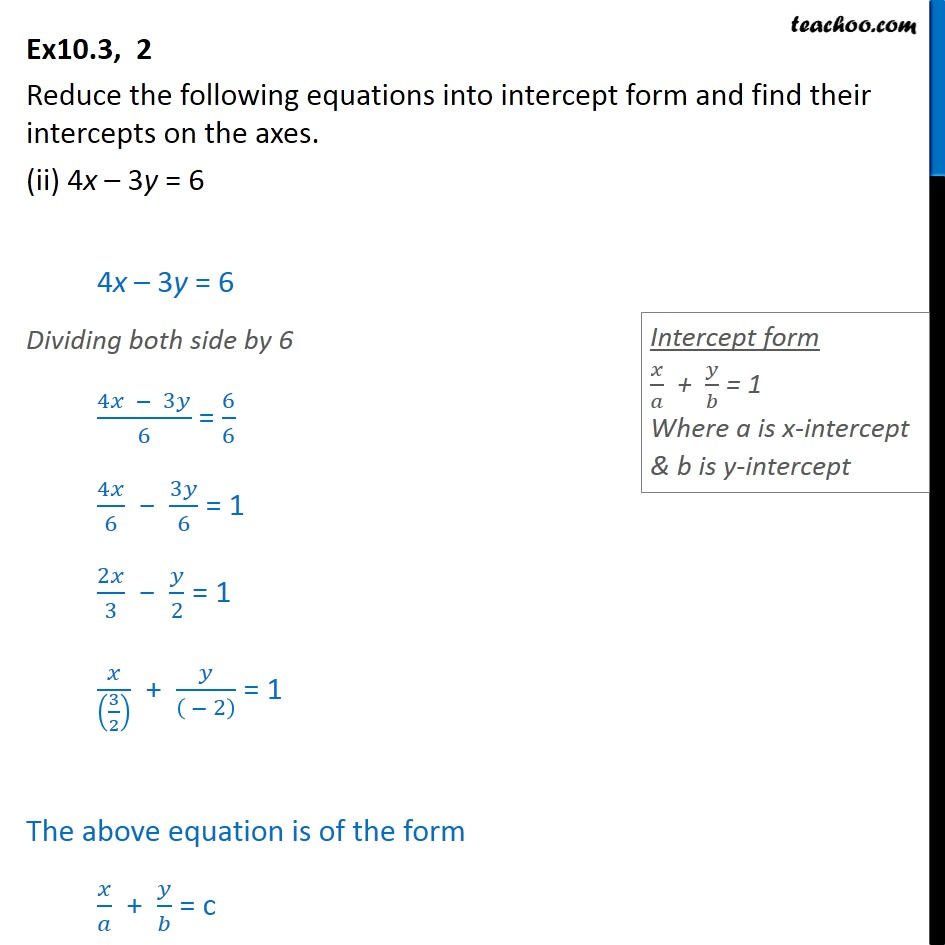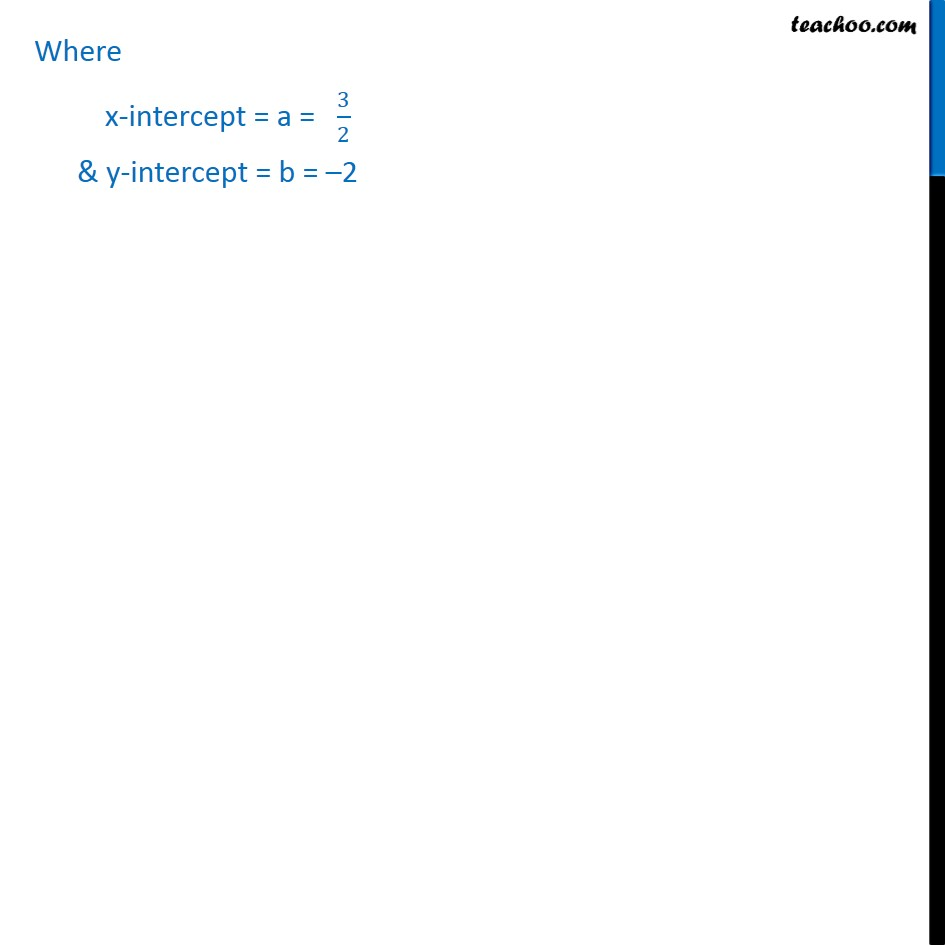Ex 9.3

Chapter 9 Class 11 Straight Lines
Serial order wiseLearn in your speed, with individual attention - Teachoo Maths 1-on-1 Class

### Transcript

Ex10.3, 2 Reduce the following equations into intercept form and find their intercepts on the axes. (ii) 4x 3y = 6 4x 3y = 6 Dividing both side by 6 (4 3 )/6 = 6/6 4 /6 3 /6 = 1 2 /3 /2 = 1 /((3/2) ) + /(( 2) ) = 1 The above equation is of the form / + / = c Where x-intercept = a = 3/2 & y-intercept = b = 2